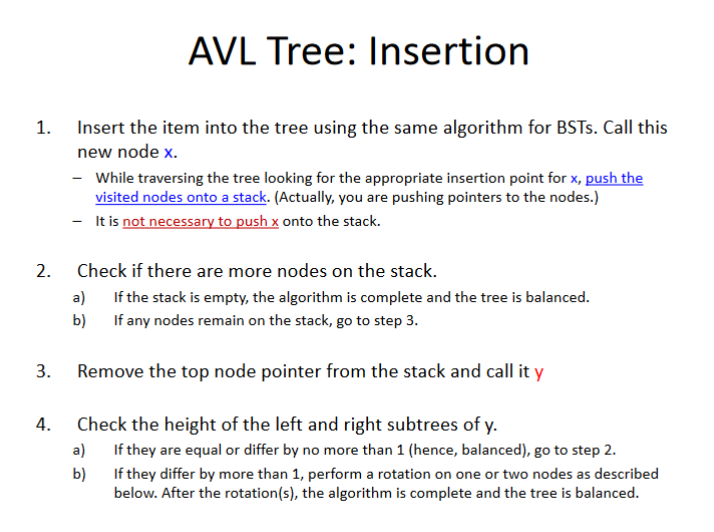# CS280 - Midterm Notes

Notes by Brandon Yu (brandon.yu@digipen.edu)

DISCLAIMER: It should be obvious, but Prahbu’s notes should always have higher priority than these notes. These should purely be supplementary to the slides. When in doubt, assume that I’m wrong.

# CS280 - Part 1 (Introductory Information)

### Introduction

Teacher: Prabhu (prabhu.n@digipen.edu)

#### General Notes

• First assignment is fucked, afterwards it’s not so fucked
• Recommended book, but not really needed: Algorithms in C++, Parts 1-4: Fundamentals, Data Structure, Sorting, Searching, Third Edition, by Robert Sedgewick
• 6 Programming Assignments (2 weeks each)
• Probably about 6 quizzes, as well
• No 60% average between midterms and finals

#### Grade Distribution:

• Programming Assignments: 35%
• Midterm exam: 20%
• Final exam: 30%
• Quizzes: 15%

#### Workload

• You will be expected to spend 5 hours/week working on assignments and 2 hours/week reviewing, reading and studying for the weekly materials. This is not including class time.
• You will receive an assignment every two weeks and have about 14 days to complete each assignment.

#### What to expect?

• Classical Abstract Data Types (ADTs): stacks, queues, trees, graphs, etc
• Basic algorithm analysis: complexity representations
• Low level implementation (in code)

#### Simple introduction to Data Structures

Data structures are a particular way of organizing data in a computer so that it can be used efficiently (Wikipedia).

• The differences between data structures is:
• The order of data in the structures
• The operations that can be used on the data structures
• The memory organization and usage of the data structure.

#### Simple introduction to Algorithms

• An algorithm is a process organized in a set of steps that mean to solve particular problems.
• Data structures support algorithms.
• Each algorithm has its pros and cons and are best used on certain types of data structures and scenarios.

#### What this course is all about

What we need to know about structures and their use in algorithms:

• The similarities
• The pros and cons of each
• Time and Space (memory) trade-off

### 3 Examples of Data Structures

#### Queue

One of the simplest data structures, the queue has a beginning and end, called the front and back of the queue.

Data enters in one end, goes out the other, like a FIFO structure, or like a checkout line in a supermarket.

Contiguous.

Bad for sorting - think about how you have to move through each element in an array.

#### Binary Tree

A Binary Tree is organized like an upside down tree. Topmost (first node) is the root node. Pointer to the root node represents the tree.

Each “node” on the tree has three things:

1. Data
2. “Left” pointer
3. “Right” pointer

Non-contiguous.

Very good for sorting (“Left” can be “smaller” and “Right” can be “larger”).

As a result, a binary tree has no duplicates, which is fine for its usage as an indexing tool. The exception is for when you have a “counter” to count duplicate values, but that’s one extra data type in each node.

#### Linked List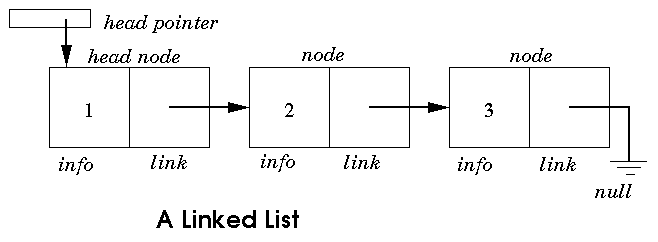You know what a linked list is.

Each node has data and a pointer to the next node. Last one has a pointer to null. Super simple.

Non-contiguous. (Obviously)

Food for thought - what happens when you delete a node in a linked list (have the previous one point to the next next one?) In an array, deleting something would result in an empty space (if you want to do it “efficiently”), but for a linked list it is easy to “reduce” the total amount of memory used.

### Locality of Reference

• Temporal Locality: Memory address which is used is likely to be used again. (Time)
• Spatial Locality: Memory addresses that are close to the ones previously used will be likely to be used again (“Space”, as in, Memory)

### Custom Memory Allocators

#### Why use a custom allocator?

1. Low usage new/delete
2. Data structures -> Linked lists, Trees, Stacks, etc. These can be used to organize your memory.
3. Solve specific constraints that are related to application.

#### Linear Allocator:

• Allocate(size) -> allocates a certain portion of the memory
• Free() -> frees entire page/block, a huge drawback.

#### Stack Allocator:

• Record the size of the block allocated in the header - we know how much is allocated per block, so we can free each block separately.
• Disadvantage - has to follow last in first out order.
• Allocate (size) -> allocate block at the top of the stack
• Free (obj * ) -> free only based on LIFO

#### Pool Allocator:

• The one done in the assignment, with pages and blocks.
• One linked list stores the page list, one more stores the free list. The “linked list” utilizes the first “block” in the memory for each node as the pointer to the next, for both page and free list. The “free” objects are then reused with a pointer in the starting block.
• Can allocate or deallocate a particular location.

#### Free List Allocator:

• Like Pool Allocator, except the size of each block is now variable
• Size of the block, obviously, is kept within the header for each block.
• When an object’s memory is freed, free list uses memory coaelescing - Free memory that is adjacent will be merged together
• Free List works the same way, which leads into the next point:

Should Allocate(size) use best fit, first fit, next fit or worst fit?

• Let’s say you have a free list of 32 bytes, 64 bytes then 16 bytes. You’re trying to fit in 10 bytes.
• First fit means it just uses 32 bytes - wastes space. There’s still 22 bytes left over. There’s a lot of fragments in your first block, usually.
• Worst fit would allocate the block to 64 bytes.
• Best fit is a size-ordered free block chain, which would allocate the block to 16 bytes. This chain needs to be sorted every single time a memory block is used.
• NEXT fit would just pick the next one starting from the first. If there is no space it will either go to the next available block or throw an exception. Then it would just go back to the first one and use the leftover memory from when it first used it.
• Buddy system is just a continual memory segmentation. It is basically best fit but with continual memory segmentation. Buddy systems are not tested.

All this so far has been external fragmentation. (Maybe? Unclear, have to do research.)

### Internal Fragmentation.

• Let’s say we have contiguous memory divided into 4kb pages.
• Process A requests 5 kb block.
• 5 KB is used by process A so 2 4kb pages are actually allocated, even though process A is only using 1kb of one of them!!

TODO: RESEARCH INTO INTERNAL AND EXTERNAL FRAGMENTATION - STILL UNCLEAR.

### Alignment

Assuming we have no alignment:

struct Student1{ int Age; //4 bytes char I1; //1 byte char I2; // 1 byte}

Student1 * pS = new Student1;

//Let's say each word is 4 bytes.
//If I ask for pS.Age, 1 operation is done.ps.I1, 1 operation is also done.pS.Age, first two bytes of age is in second word, last two bytes of age are in third word. So 2 operations are required - second AND third words have to be accessed.

struct Student1{ char I1; //1 byte int Age; //4 bytes char I2; // 1 byte}

pS.Age -> 2 operations
pS.I1  -> 1 operation
pS.I2  -> 1 operation
pS.Age -> 2 operations

Solution:

• Align the data with the memory addresses.
• How?
• Padding! The normal method for padding for each obj in the struct would be to pad to the nearest word size.
• Note that this may not be true – but that’s the system Prahbu is using for this class.

### Algorithm Analysis

Variables important to analyzing algorithms:

• Correctness
• Complexity
• Time
• Space

### Search Algorithms

• Just go one by one.
• Worst case scenario: requires n readings for an array of n size (if the element is at the end)
• Linear-Time
• The elements are in sorted order
• If you want to find a particular element “3” in “1,2,3…10”, get the middle (0+(n-1))/2 = (0+9)/2=4
• Search in the left sub-array first (because 3 is less than 5, which is arr)
• Keep going
• Logarithmic-Time (Time is proportional to the logarithm of the size of the problem)

### Big-O Notation

Big-O Notation assumes the worst (longest) asymptotic computational time. So, in a conditional, it will go with the worst case every time.

For Big-O Notation, all operations take a constant time. Big-O Notation is a theoretical analysis, and does not actually glean the actual computing time required for the operation.

The dominant term is the term the one that gets biggest (i.e. dominates) as N gets bigger. So in O(n^2+2n+100), n^2 is the dominant term. An easy way to think about it is that if you make N go towards infinity, what encapsulates the vast majority of the computation time? That is the dominant term.

### Examples of Big-O Notation Comparisons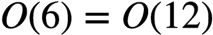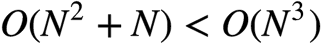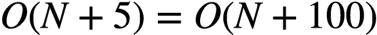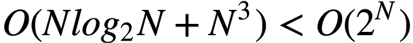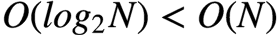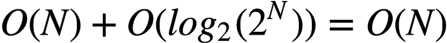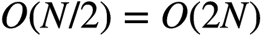(For the following, left hand side is log10N/log10e, latter of which is a constant)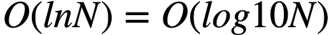If you get confused, just assume n is some constant like 10 and try to calculate the value…

#### Important Notations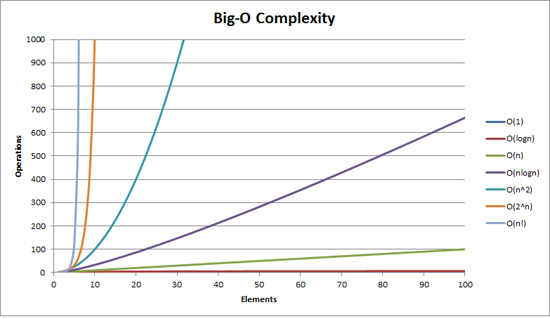##### O(1)

O(1) describes an algorithm that will always execute in the same time (or space) regardless of the size of the input data set.

##### O(N)

O(N) describes an algorithm whose performance will grow linearly and in direct proportion to the size of the input data set. The example below also demonstrates how Big O favours the worst-case performance scenario; a matching string could be found during any iteration of the for loop and the function would return early, but Big O notation will always assume the upper limit where the algorithm will perform the maximum number of iterations.

##### O(N^2)

O(N^2) describes an algorithm whose performance will grow linearly and in direct proportion to the size of the input data set. The example below also demonstrates how Big O favours the worst-case performance scenario; a matching string could be found during any iteration of the for loop and the function would return early, but Big O notation will always assume the upper limit where the algorithm will perform the maximum number of iterations.

bool ContainsDuplicates(IList<string> elements)
{
for (var outer = 0; outer < elements.Count; outer++)
{
for (var inner = 0; inner < elements.Count; inner++)
{
// Don't compare with self
if (outer == inner) continue;
if (elements[outer] == elements[inner]) return true;
}
}
return false;
}

##### O(2^N)

O(2^N) denotes an algorithm whose growth doubles with each additon to the input data set. The growth curve of an O(2^N) function is exponential - starting off very shallow, then rising meteorically. An example of an O(2N) function is the recursive calculation of Fibonacci numbers.

int Fibonacci(int number)
{
if (number <= 1) return number;
return Fibonacci(number - 2) + Fibonacci(number - 1);
}


#### Fastest to Slowest

FASTER (less dominant)

O(1)
O(log n)
O(n)
O(n log n)
O(n^2)
O(n^3)
O(n^k) - Polynomial
O(2^n) - Exponential

SLOWER (more dominant)

#### Chaining together Big-O Notation

g()                      <- O(N)
for (j=0;j<N;++j) g(j)   <- O(N^2)

#### Formal approach

• Size of the problem
• Constant
• Variable
• Combination

Theres Big-Oh, Big-Theta, Big-Omega, but the latter two are described in CS330, not here.

### Wikipedia Big-O Complexity Listings

Note that for this list, it’s specifically talking about when you have a subscript.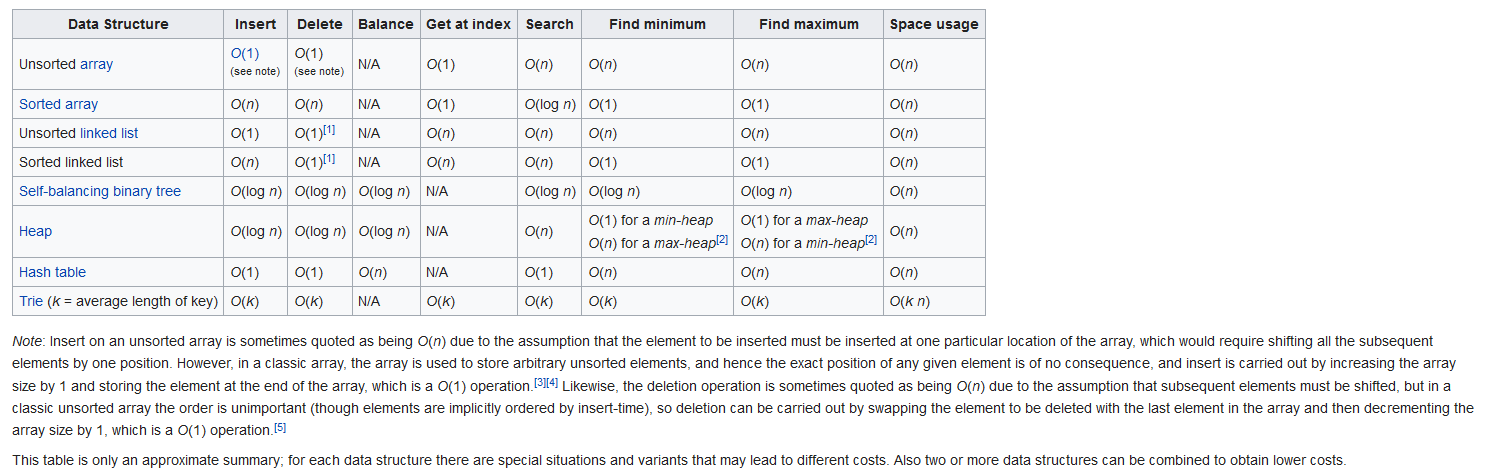### Big-O Cheat Sheet (Important!!!)

Great website suggested by Louis:
http://bigocheatsheet.com/

# CS280 - Part 2 (Common Sorting Algorithms)

## Introductory Sorting - Part 1

### Big-O Complexity Cheat Sheet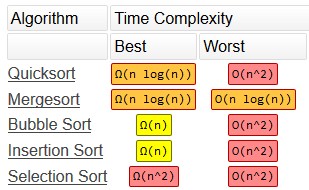### Overview and Memorization Techniques

Note: These are just methods I use to memorize. Feel free to ignore this section.

Bubble Sort: Elements “bubble” to the top of the list, by continuous comparisons and swaps. “Bubbles” on the top are regarded as sorted.
Selection Sort: Two groups, sorted and unsorted. The smallest from the unsorted (which is still bigger than the largest in the sorted) is selected and put in the sorted at the end of the sorted group.
Insertion Sort: Two groups, sorted and unsorted. The first in the unsorted is inserted into the sorted group.
Merge Sort: Every element is an individual group at the start. Sorted elements are merged then sorted.
Quick Sort: I dunno how the fuck to memorize this one easily. gg.com.sg

### Sorting

Example of the usage of a sorting algorithm:

a1 -> a2 -> a5 -> a3 -> a4

Goes through algorithm (Sort)

a1-> a2 -> a3 -> a4 -> a5


### Attributes of Algorithms:

1. Stable/Unstable
• Preserves the relative order of items with equal values.
• 7,5,6,5,2 -> 2,5,5,6,7
• Which 5 goes in front of which? If it cares, it’s stable, otherwise it is not stable.
2. In-place / Out-of-place sorting
• If you have an array of 5 integers and your algorithm uses exactly the same amount of memory, it is in-place sorting, otherwise it is out-of-place sorting.
• If the memory usage is constant even with increasing amounts of elements in the container, then it is in-place. Otherwise, it is out-of-place.
• A convenient example is swapping.
3. Adaptive
• If the array is sorted partially already and the algorithm takes advantage of this, it’s adaptive.
4. Loop Invariant (Not gone much into in this course, probably not even tested)
• Loop invariant is a property that holds before (and after) each repetition.
• For example, in this, it is true that (for every iteration), i+j==9. A weaker invariant that is also true is that i >= 0 && i < 10 (because this is the continuation condition!) or that j <= 9 && j >= 0.
int j = 9;
for(int i=0; i<10; i++)
j--;


### Sorting Algorithms

https://visualgo.net/bn/sorting is extremely useful for visualization of the different sorts.

#### Bubble Sort

##### Explanation

Assume an input array of

5 3 8 4 6
^ ^


First, compare the ith element and the (i+1)th element.

5 is more than 3, so let’s do a swap.

3 5 8 4 6
^ ^


Keep increasing i, iterating through…

3 5 8 4 6
^ ^

3 5 4 8 6
^ ^

3 5 4 8 6
^ ^

3 5 4 6 8 <- Note the highest number is in the last place.


That’s one pass.

Now the highest element (8) is in the correct position, at the end. So we ignore it on the next pass. Similarly, on the next pass we ignore the second last, and so on.

Keep doing passes until the array is sorted.

##### C++ Implementation
###### Non-Adaptive Implementation
void bubbleSort(int arr[], int n)
{
int i, j;
for (i = 0; i < n-1; i++)
// Last i elements are already in place
for (j = 0; j < n-i-1; j++)
if (arr[j] > arr[j+1])
swap(&arr[j], &arr[j+1]);
}

###### Adaptive Implementation
void bubbleSort(int arr[], int n) {
bool swapped = true;
int j = 0;
int tmp;

while (swapped) {
//Note the boolean - it's important in this adaptive implementation
swapped = false;
j++;
for (int i = 0; i < n - j; i++) {
if (arr[i] > arr[i + 1]) {
tmp = arr[i];
arr[i] = arr[i + 1];
arr[i + 1] = tmp;
swapped = true;
}
}
}
}

##### Big-O Complexity
Worst case: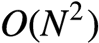Best case :
(Doesn’t really do the inside loop since it always goes into the else in the if conditional)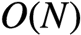##### Attributes
• Stable
• In-place
• Non-adaptive or Adaptive depending on implementation - one way to make it adaptive is to use a boolean to check if a swap has to be done, in which case it’s adaptive)

#### Selection Sort

##### Explanation

(From Wikipedia)

The algorithm divides the input list into two parts: the sublist of items already sorted, which is built up from left to right at the front (left) of the list, and the sublist of items remaining to be sorted that occupy the rest of the list. Initially, the sorted sublist is empty and the unsorted sublist is the entire input list. The algorithm proceeds by finding the smallest ((or largest, depending on sorting order) element in the unsorted sublist, exchanging (swapping) it with the leftmost unsorted element (putting it in sorted order), and moving the sublist boundaries one element to the right. 

##### C++ Implementation
/* a to a[n-1] is the array to sort */
int i,j;
int n;

/* advance the position through the entire array */
/*   (could do j < n-1 because single element is also min element) */
for (j = 0; j < n-1; j++)
{
/* find the min element in the unsorted a[j .. n-1] */
/* assume the min is the first element */
int iMin = j;
/* test against elements after j to find the smallest */
for (i = j+1; i < n; i++) {
/* if this element is less, then it is the new minimum */
if (a[i] < a[iMin]) {
/* found new minimum; remember its index */
iMin = i;
}
}

if(iMin != j)
{
swap(a[j], a[iMin]);
}
}

##### Big-O Complexity
Best-case and worst-case are both:##### Attributes
• Unstable
• In-place
• Non-adaptive (Worst-case and best-case are the same)

#### Insertion Sort

##### Explanation

From Wikipedia:

Insertion sort iterates, consuming one input element each repetition, and growing a sorted output list. At each iteration, insertion sort removes one element from the input data, finds the location it belongs within the sorted list, and inserts it there. It repeats until no input elements remain.

Sorting is typically done in-place, by iterating up the array, growing the sorted list behind it. At each array-position, it checks the value there against the largest value in the sorted list (which happens to be next to it, in the previous array-position checked). If larger, it leaves the element in place and moves to the next. If smaller, it finds the correct position within the sorted list, shifts all the larger values up to make a space, and inserts into that correct position.

Main Idea: Keep inserting next element into the sorted sequence.

1. Get the 1st element from the unsorted side.
2. Find the correct position on the sorted side.
3. Insert the element into the sorted side.
##### C++ Implementation
void InsertionSort (int a[], int N) //a is array, N is size of said array.
{
for(int i=1;i<N;++i)
{
int j=i; //Get first element from the unsorted side.
int current = a[i]; //Set current variable to the first element value.

//Find the correct position to insert into.
while((j>0) //While j is still within bounds and
&& (a[j-1]>current)) //The current value is more than the current
{
a[j]=a[j-1];
--j;
}

//Insert.
a[j] = current;
}
}
}

##### Big-O Complexity
Best-case, if sorted, the while loop doesn’t execute, soWorst case, with the while loop constantly being called with tons of steps,Explanation for worst case is, the max amount of times you may need to step through is:In terms of Big-O Notation,##### Attributes
• Stable (Doesn’t change the order of duplicates)
• In-place
• Adaptive

#### Merge Sort

##### Recursion

So a quick primer on recursive functions. When doing recursive functions, the parameter and signature of the function call will be added to said stack (called activation records, which are then executed in reverse order).

In other words, you’re fucking up your call stack and by extension, your memory usage. You should know this. For recursive functions, the Big-O Complexity required can be calculated by splitting the recursive function into separate recursive functions.

Reason this is relevant is that Merge Sort primarily uses recursion to sort.

##### Explanation

Merge Sort is basically just the two steps repeated continuously.

1. Divide
2. Merge

To be more precise, we first divide the array into its elements. Then we merge them together into pairs in the correct order.

We start with

38 2 43 3        < Input

We divide them into 2 sections...

38 27       43 3 < Input

Sort each section using the below method (It's clearer later)

27 38       3 43 < Input

Okay, now choose smallest from the 2 lowest, 27 and 3.

3 is the smallest, so we strike that off the list.

27 38         43 < Input
3                < Output

Now we pick between 27 and 43.

38            43 < Input
3 27             < Output

Do it again.

43               < Input
3 27 38          < Output

Finally...

3 27 38 43       < Output
##### Pseudo-Code
split each element into partitions of size 1

recursively merge adjancent partitions

for i = leftPartStartIndex to rightPartLastIndex inclusive

if leftPartHeadValue <= rightPartHeadValue

copy leftPartHeadValue

else: copy rightPartHeadValue

copy elements back to original array
##### C++ Implementation
void DoMergeSort ( int a[], int left, in right) {
if(left<right) {
unsigned const middle = (left+right)/2;
DoMergeSort(a, left, middle)
DoMergeSort(a, middle+1, right);
Merge(a, left, middle, right);
}
}

void Merge(int array[], int left, int middle, int right){ //right is the location of the end element of the right array, and left is the location of the end location of the left array, NOT one past the last.
unsigned i = left; // counter for the temp array
unsigned j = left; // counter for left array
unsigned k = middle + 1; // counter for right array
int* temp = new int [right+1];
while (j<=middle && k <=right)
if (array[j] <= array[k])
temp[i++] = array[j++];
else
temp[i++] = array[k++];
//Handle the leftovers, one of these two will be excuted.
while (j <= middle)
temp[i++] = array[j++];
while (k <= right)
temp[i++] = array[k++];
//Copy from temp array to input array.
for (i=left; i <= right; ++i)
array[i] = temp[i];
delete [] temp;
}

##### Big-O Complexity

Complexity calculation:Best Case: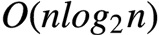Worst Case:#### Quick Sort

##### Explanation

Quicksort is a divide and conquer algorithm. Quicksort first divides a large array into two smaller sub-arrays: the low elements and the high elements. Quicksort can then recursively sort the sub-arrays.

The steps are:

1. Pick an element, called a pivot, from the array.

2. Partitioning: reorder the array so that all elements with values less than the pivot come before the pivot, while all elements with values greater than the pivot come after it (equal values can go either way). After this partitioning, the pivot is in its final position. This is called the partition operation.

3. Recursively apply the above steps to the sub-array of elements with smaller values and separately to the sub-array of elements with greater values.

The base case of the recursion is arrays of size zero or one, which never need to be sorted.

The pivot selection and partitioning steps can be done in several different ways; the choice of specific implementation schemes greatly affects the algorithm’s performance.

##### Pseudocode

(From VisualAlgo)

for each (unsorted) partition

set first element as pivot

storeIndex = pivotIndex + 1

for i = pivotIndex + 1 to rightmostIndex

if element[i] < element[pivot]

swap(i, storeIndex); storeIndex++

swap(pivot, storeIndex - 1)
##### Big-O Complexity
Best case:
When pivot is continuously swapped to the center of the array. After partitioning, it should be in the middle, with the neighbouring partitions being of equal size.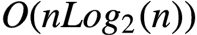Worst case:
When the array is already sorted (in both ways). The size of one of the neighbouring partitions to the pivot at the end will be 0. This is called an unbalanced split.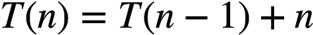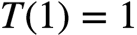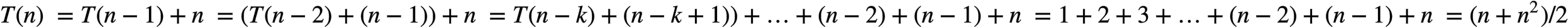So, worst case is…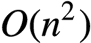# CS280 - Part 3 (ADTs) (Unfinished)

## Abstract Data Types

### Introduction

An Abstract Data Type is a data type (a set of values and a collection of operations on those values) that is accessed only through an interface (Hence, abstract, since functionality is hidden).

We refer to a program that uses an ADT as a client and a program that specifies the data type as an implementation.

### Advantages

1. Code re-use/Modular programming
2. Easy and robust testing
3. Change the functionality without change in the client code

### Stack Implementation

• Push
• Pop

#### Interface:

1. Stack(int capacity) //constructor
2. void Push (char item)
3. char Pop(void)
4. IsEmpty(void)

#### Complexity for push and pop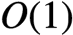If you want to grow your stack size and it’s not a linked-list stack, then it’s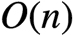TODO

TODO

TODO

# CS280 - Part 4 (BSTs)

## Tree

Fast insertion/deletion/search.

## Big-O Complexity

Search is: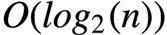## Terminology

Nodes are also known as vertexes.
An Edge is the connection (line) between one node to another.

Root node is the first node.
Leaf nodes are nodes without any children.
Parent node and Child node are also common terminology, but should be obvious.
Sibling nodes are nodes that share a common parent.
There’s also uncle nodes and grandfather nodes and grandgrandfather nodes but those aren’t gone into much detail.

## Height

Height of a tree is the “levels”, but not including a root node. (The level for just a root node is considered to be 0)

## Sub-trees

Sub-tree is a part of a tree including that node and its children and its childrens’ children, and so on.

## Ordered and unordered Trees

Binary tree is at most 2 child nodes. (One is called left child node, one is called right child node) (Can have ONE child node)

M-ary Tree can be any number of child nodes.

## Proprties

1. Only 1 parent per node.

2. No cycles in the tree (no closed loops in the tree.) (Usually this breaks the above rule anyway)

3. No multiple paths from one node to another node.

4. Root node to leaf node there should be only one path

5. Level = 0, if it is root node. Level = Level (parent)+1, if it is a child node of some parent node.

## Balanced Binary Tree

Balanced in terms of height.

Balance factor

The constraint is generally applied recursively to every subtree. That is, the tree is only balanced if:

1. The left and right subtrees’ heights differ by at most one, AND
2. The left subtree is balanced, AND
3. The right subtree is balanced

According to this, the next tree is balanced:

     A
/   \
B     C
/     / \
D     E   F
/
G  

The next one is not balanced because the subtrees of C differ by 2 in their height:

     A
/   \
B     C   <-- difference = 2
/     /
D     E
/
G  

That said, the specific constraint of the first point depends on the type of tree. The one listed above is the typical for AVL trees.

Red-black trees, for instance, impose a softer constraint.

## Full Binary Tree

A Binary Tree is full if every node has either 0 or 2 child nodes.

## Complete Binary Tree

• Balanced.
• Every node except the last level’s nodes have 2 child nodes (each level except the last level being completely filled), and they are filled as far left as possible.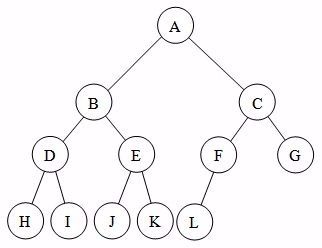## Trees vs Linked List

Trees have “left and right”, linked list is “previous and next”.

## Order of Traversal

A is parent, B is left child, C is right child.

Different orders of traversal are useful for different sorting methods.

### In-Order

B,A,C

Algorithm In-order(tree)

1. Traverse the left subtree, i.e., call Inorder(left-subtree)
2. Visit the root.
3. Traverse the right subtree, i.e., call Inorder(right-subtree)

### Pre-Order

A,B,C

Algorithm Pre-order(tree)

1. Visit the root.
2. Traverse the left subtree, i.e., call Preorder(left-subtree)
3. Traverse the right subtree, i.e., call Preorder(right-subtree)

### Post-Order

B,C,A

Algorithm Postorder(tree)

1. Traverse the left subtree, i.e., call Postorder(left-subtree)
2. Traverse the right subtree, i.e., call Postorder(right-subtree)
3. Visit the root.

### Example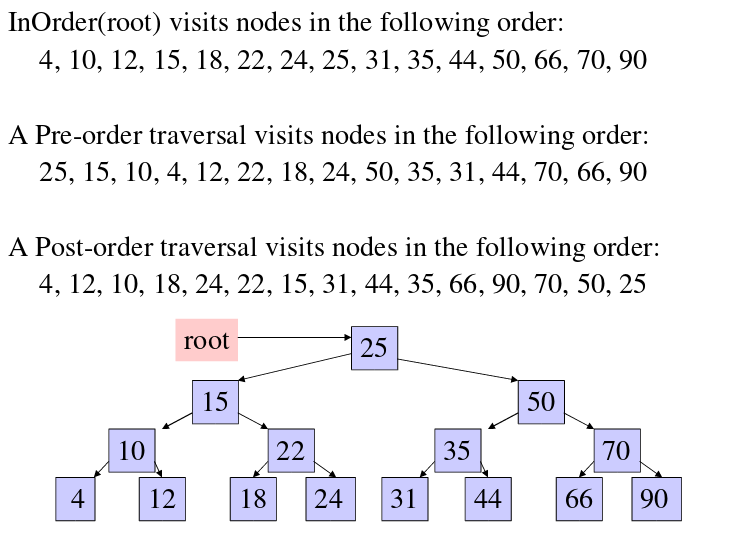## Binary Search Tree / Binary TreesA Binary Search Tree (BST) is a tree in which all the nodes follow the below-mentioned properties −

    The left sub-tree of a node has a key less than or equal to its parent node's key.

The right sub-tree of a node has a key greater than to its parent node's key.

Thus, BST divides all its sub-trees into two segments; the left sub-tree and the right sub-tree and can be defined as −

left_subtree (keys) ≤ node (key) ≤ right_subtree (keys)

Max number of nodes in BST is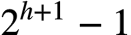External Nodes or Terminal Nodes are Leaf Nodes

Everything else is an internal node.

A binary tree with N internal nodes has N+1 external nodes (some may be empty/NULL).

A binary tree with N internal nodes has 2N links.

Does not have duplicate values.

A binary tree does not have to be balanced.

### Big-O Complexity

#### Worst case

When the data is skewed all the way to left or right.#### Best case

Every other case: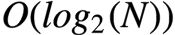#### For Deletion

4 Cases:

1. Node x is a leaf node,.
Set parents pointer to this node to be null Release the memory of the node. 
2. Node x has an empty left child.
3. Node x has an empty right child.
Replace the node's data (including pointers) with the left/right child. Then delete the child's memory. 
4. Node x has both left and right children.

4 is trickiest. To solve it, we need the predecessor of the node, which is just going to be the largest node in its left sub-tree

Then, replace node X’s data with the predecessor’s data (NOT the pointers)

Then delete that node. Try to use the previous cases from there.

### Promotion/Rotation

Promotion and rotation are the same thing.

https://en.wikipedia.org/wiki/Tree_rotation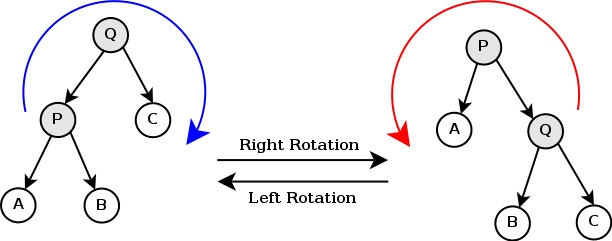In the above example, right rotation is P being promoted (going “up”)

# CS280 - Part 5 (AVL Trees)

## Intro

AVL Trees are notable for being the first trees to have worst search time ofAVL Tree is a balanced BST. For all nodes in the BST:

| Height of left sub tree - Height of right subtree | = 1 or 0.

## Insertion

http://www.geeksforgeeks.org/avl-tree-set-1-insertion/